# Atoms and Molecules

## Laws of Chemical Combination

There are two main laws of Chemical Combination as established by Lavoisier and Joseph L. Proust.
(a)Law of Conservation of Mass
(b) Law of constant or definite proportion

### Law of Conservation of Mass

It states that mass cannot be created or destroyed in chemical reaction. So mass of reactants is mass of the Product.

A + B -> C +D
Mass of Reactants=Mass of (A+B)
Mass of Products = Mass of (C+D)
Mass of Reactants = Mass of Products

### Law of constant or definite proportion

It states that elements combine in their definite proportion of mass to give compounds. or In a chemical substance the elements are always present in definite proportions by mass
Example
Compound $CO_2$ can be obtained using various ways
$C + O_2 -> CO_2$
$CaCO_3 -> CaO + CO_2$
The ratio of Carbon and Oxygen is always same ie. 12:32
Similarly For Water $H_2O$, the ratio of the mass of hydrogen to the mass of oxygen is always 1:8, whatever the source of water

## Dalton's atomic theory

There was explanation for the above theories.
British chemist John Dalton proposed the atomic theory which provided the explanation for the above laws
• All matter is made of very tiny particles called atoms.
• Atoms are indivisible particles
• Atoms can neither be created nor be destroyed
• Atoms of same elements are similar.
• Atoms of different elements are different.
• Atoms combine in the ratio of small whole numbers to form compounds.
• The relative number and kinds of atoms are constant in a given compound.

## What is an Atom

• Matter is made up of Atoms
• Atom are the smallest particle of elements
• Atoms are very small, they are smaller than anything that we can imagine or compare with
• The size of the atoms is measured by the Atomic Radius .Atomic radius is measured in nanometres ( $1 \ nm= 10^{-9} \ m$)
• Atomic Radius of the Hydrogen is $10^{-10} \ m$. And it is the smallest of all

## Symbols of Atoms or Elements

• Dalton proposed the below symbols for the atoms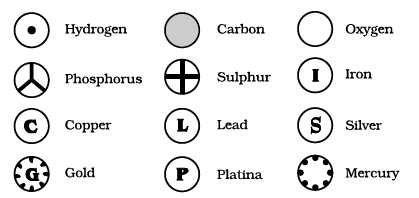• Berzilius suggested that the symbols of elements be made from one or two letters of the name of the element.
• Now-a-days, IUPAC (International Union of Pure and Applied Chemistry) approves names of elements
• Now generally symbols are the first one or two letters of the element's name in English. The first letter of a symbol is always written as a capital letter (upper-case) and the second letter as a small letter (lower-case).Symbols of some elements are formed from the first letter of the name and a letter, appearing later in the name
Example
Hydrogen - H
cobalt - Co
Chlorine - Cl
• There are few elements where the symbols were taken from names of element in Latin ,German & Greek
Example
Fe from its Latin name ferrum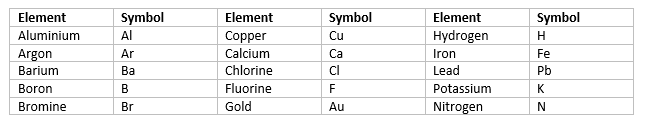## Atomic Mass

• Each element had a characteristic atomic mass
• The mass of one atom is called as atomic mass
• We define One atomic mass unit is a mass unit equal to exactly one-twelfth (1/12th) the mass of one atom of carbon-12
• relative atomic masses of all elements have been found with respect to an atom of carbon-12.
• So, Atomic mass of atom is measured in amu. amu is written as 'u' � unified mass as per latest IUPAC recommendations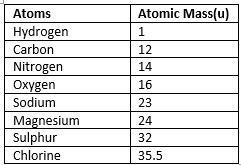## What is Molecule

• A molecule is a group of two or more atoms chemically bonded together. The atoms with in the molecules are held of force of attraction.
• It is smallest particle of an element or a compound that is capable of an free state and that has all the properties of that substance.
Molecules of Elements
-Generally atoms of most of the elements exists as molecules Like Oxygen exists as $O_2$, Hydrogen exists as $H_2$. The number of atoms constituting a molecule is known as its atomicity

Molecules of Compounds
- The molecule of compounds contains two or more different atoms chemical bonded together.
Example
$HCl$
$H_20$

## Ions

• Compounds composed of metals and non-metals contain charged species
• The charged species are known as ions.
• An ion is a charged particle and can be negatively or positively charged.
• Anions are negatively charged ion while cations are the positively charged ion
• Anions are formed by gain of electrons while cations are formed by loss of electrons
• example Compound Sodium chloride NaCl consists of Positively charged $Na^+$ and negatively charged ion $Cl^{-}$
• An Ion can have multiple atoms which has net charge on it. These are called polyatomic ions

## Valency

The combining power (or capacity) of an element is known as its valency. For ions, the charge indicates the valency of the ions

## Chemical Formulae

The chemical formula of a compound is a symbolic representation of its composition. The chemical formula can be written based on these information
(a) Symbols of the elements involved
(b) The valency of the elements or ions and this must be balanced in formula
(c) when a compound consists of a metal and a non-metal, the name or symbol of the metal is written first
(d) in compounds formed with polyatomic ions, the ion is enclosed in a bracket before writing the number to indicate the ratio. In case the number of polyatomic ion is one, the bracket is not required.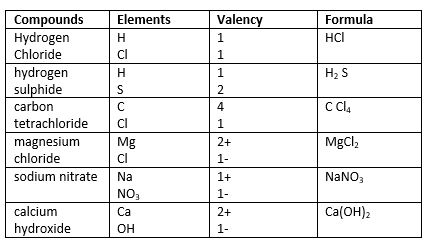## Molecular Mass

The molecular mass of a substance is the sum of the atomic masses of all the atoms in a molecule of the substance.
This is also expressed in terms of u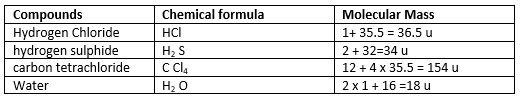## FORMULA UNIT MASS

• formula unit mass is used for those substances whose constituent particles are ions
• it is sum of the atomic masses of all atoms in a formula unit of a compound
example
NaCl
Formula Unit Mass = 23 + 35.5=58.5 u

## Mole Concept

• Wilhelm Ostwald Introduce the word "mole" in 1896 .It is derived from Latin word moles meaning a 'heap' or 'pile'
• One mole of any species (atoms, molecules, ions or particles) is that quantity in number having a mass equal to its atomic or molecular mass in grams
• The number of particles (atoms, molecules or ions) present in 1 mole of any substance is fixed, with a value of $6.022 \times 10^{23}$
• The above number is called Avogadro constant.
• The mass of 1 mole of a substance is equal to its relative atomic or molecular mass in grams. This is called Molar Mass .This is also called gram atomic mass
• So we just need to replace u in atomic mass or Molecular with gm to get the Molar mass of the substance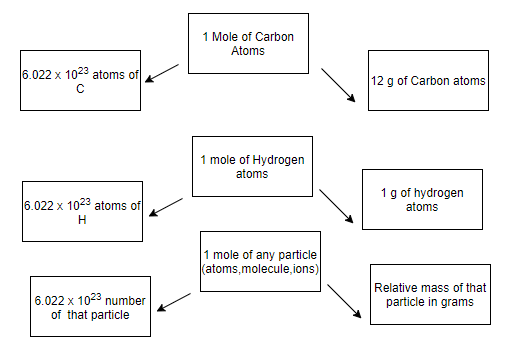Some Formulas
$mass = \text {molar mass} \times \text {number of moles}$
$\text {number of moles}= \frac {\text{The number of particles}}{\text{Avogadro number}}$
$\text {The number of atoms}= \frac {\text{given mass}}{\text{molar mass}} \times \text{Avogadro number}$
$\text {The number of Molecules}= \frac {\text{given mass}}{\text{molar mass}} \times \text{Avogadro number}$
$\text {The number of particles} =\text {number of moles of particles} \times \text{Avogadro number}$

Example
Calculate the number of particles in each of the following:
(i) 23 g of Na atoms (number from mass)
(ii) 8 g $O_2$ molecules (number of molecules from mass)
(iii) 0.1 mole of carbon atoms (number from given moles
Solution
$\text {The number of atoms}= \frac {\text{given mass}}{\text{molar mass}} \times \text{Avogadro number}$
$= \frac {23}{23} \times 6.022 \times 10^{23}$
$=6.022 \times 10^{23}$
(ii) $\text {The number of molecules}= \frac {\text{given mass}}{\text{molar mass}} \times \text{Avogadro number}$
Now molar mass of O2 molecules
= 16 x 2 = 32g
Therefore
$= \frac {8}{32} \times 6.022 \times 10^{23}$
$=1.51 \times 10^{23}$
(iii) $\text {The number of particles} =\text {number of moles of particles} \times \text{Avogadro number}$
$= 0.1 \times 6.022 \times 10^23$
$= 6.022 \times 10^{22}$

Latest Articles
Synthetic Fibres and Plastics Class 8 Practice questions

Class 8 science chapter 5 extra questions and Answers

Mass Calculator

3 Fraction calculator

Garbage in Garbage out Extra Questions7